## Fourier Cosine Series

If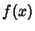is an Even Function, then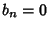and the Fourier Series collapses to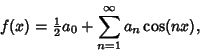(1)

where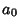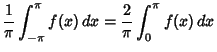(2)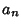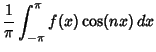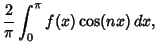(3)

where the last equality is true because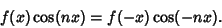(4)

Letting the range go to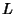,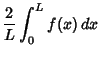(5)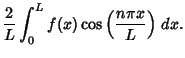(6)

See also Even Function, Fourier Cosine Transform, Fourier Series, Fourier Sine Series